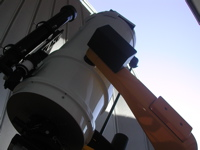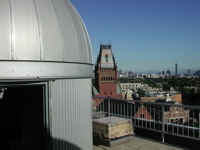# Astronomy Lab and Clay TelescopeCCD Field of View using Albireo

EL Obs 2

SPU-21 Fall 2015

printer version

Let's determine the CCD field of view! Using the known separation of the double star, Albireo (34 arcsec - given in the Visual Observations of Albireo lab), we can determine the size of the CCD in arcminutes.

Procedure:

• Slew the telescope to Albireo RA=19:30:43 DEC=+27:57:40
• Take a very short (0.1 sec) exposure, making sure not to saturate the image. If necessary, use the Aperture Mask to essentially make our telescope aperture smaller and allow a longer exposure on the CCD before it saturates.
• Load the image you took above in MaximDL
• Measure the X, Y pixel coordinates of each of the 2 stars by hovering the curser on the center of each star in turn and then recording the X,Y coordinates in the table (pdf or docx).

Analysis:

• Star 1 has coordinates (X1, Y1) and star 2 has coordinates (X2, Y2).
• Consider the difference between stars (in pixels) the distance, Z, where Z = ((X1-X2)2 + (Y1-Y2)2) 1/2 [Do you recognize this equation? The hypotenuse of a right triangle!]
• When you divide this by the separation between the stars (34 arcsec), this gives you the number of arcsec/pixel on your CCD image.
• Determine the number of pixels your CCD image contains in X, Y by roaming the curser from one side of the image to the other.
• Since you now have the conversion of arcsec/pixel, use this to find the corresponding size (in arcsec, and then convert to arcmin) of the full CCD.